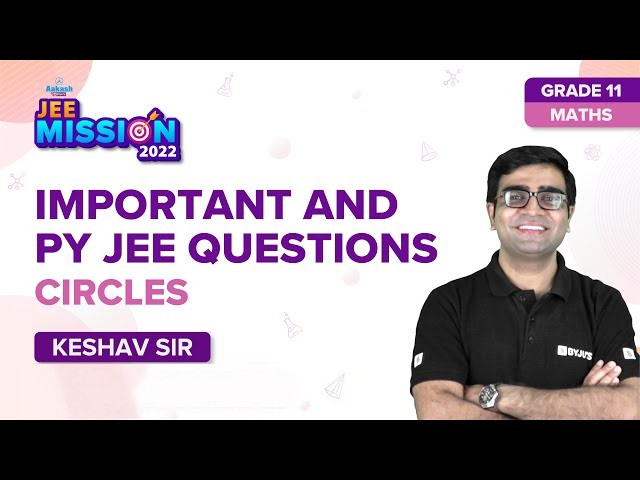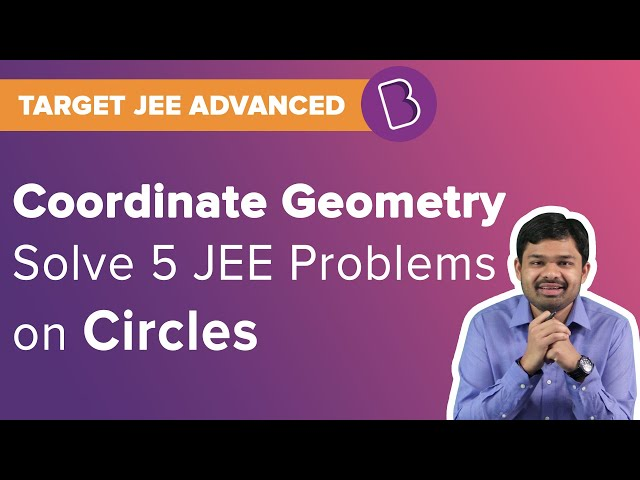# JEE Circles and System of Circles Previous Year Questions With Solutions

JEE Previous year questions with solutions on circles and system of circles are provided on this page for the JEE aspirants. Some of the topics which are covered under this chapter are the definition of a circle, general equation of the circle, equation of a circle with the endpoints of diameter, equation of a tangent to a circle, equation of normal to a circle, equation of a circle passing through a line and touching a line, length of a tangent from an external point, equation of chord and orthogonal circles.

Students can expect about 1 – 2 questions from this chapter in the JEE examination. We have also provided detailed solutions of all important previous year questions for all the individual chapters to help students in clearing their doubts that they might encounter while solving previous year questions.

## JEE Main Past Year Questions With Solutions on Circles and System of Circles

Question 1: Circles x2 +y2 – 2x – 4y = 0 and x2+ y2– 8y – 4 = 0

A) Touch each other internally

B) Touch each other externally

C) Cuts each other at two points

D) None of these

Solution:

$$\begin{array}{l}{{C}_{1}}(1,\ 2),\ {{C}_{2}}(0,\ 4),\ {{R}_{1}}=\sqrt{5},\ {{R}_{2}}=2\sqrt{5}\\ {{C}_{1}}{{C}_{2}}=\sqrt{5} \\ \ {{C}_{1}}{{C}_{2}}=\ |{{R}_{2}}-{{R}_{1}}|\end{array}$$

Hence, circles touch internally.

Question 2: The locus of the centre of a circle passing through (a, b) and cuts orthogonally to circle x2 + y2 = p2 is

Solution:

Let equation of circle be

$$\begin{array}{l}{{x}^{2}}+{{y}^{2}}+2gx+2fy+c=0\end{array}$$
with
$$\begin{array}{l}{{x}^{2}}+{{y}^{2}}={{p}^{2}}\end{array}$$
cutting orthogonally, we get
$$\begin{array}{l}0+0=+c-{{p}^{2}}\\ c={{p}^{2}}\end{array}$$
and passes through (a, b), we get

$$\begin{array}{l}{{a}^{2}}+{{b}^{2}}+2ga+2fb+{{p}^{2}}=0\\ 2ax+2by-({{a}^{2}}+{{b}^{2}}+{{p}^{2}})=0\end{array}$$

Required locus as a centre (−g, −f) is changed to (x, y).

Question 3: The equation of the line passing through the points of intersection of the circles 3x2 + 3y2 – 2x + 12y – 9 = 0 and x2 + y2 + 6x + 2y – 15 = 0 is

Solution:

Let S1 = 3x2 + 3y2 – 2x + 12y – 9 = 0

divide by 3, we get S1 = x2 + y2 – (2/3)x + 4y – 3 = 0

S2 = x2 + y2 + 6x + 2y – 15 = 0

Common chord  = S1 – S2

=> 10x – 3y – 18 = 0.

Question 4: If two circles (x-1)2 + (y-3)2 = rand x2 + y2 – 8x + 2y +8 = 0 intersect in two distinct points, then

(a) 2 < r< 8

(b) r = 2

(c) r < 2

(d) r > 2

Solution:

When two circles intersect each other, then

Difference between their radii < Distance between centres

⇒ r − 3 < 5 ⇒ r < 8 …..(i)

Sum of their radii > Distance between centres …..(ii)

⇒ r + 3 > 5 ⇒ r > 2

Hence by (i) and (ii), 2 < r < 8.

Hence option a is the answer.

Question 5: The length of the chord joining the points in which the straight line (x/3) +(y/4) =1 cuts the circle x2+ y2 =169/25 is

A) 1

B) 2

C) 4

D) 8

Solution:

Length of the perpendicular to the line 4x + 3y = 12 from (0, 0) =12/5

Radius of the circle is 13/5.

Required length

$$\begin{array}{l}=2\sqrt{\frac{169}{25}-\frac{144}{25}}=2\end{array}$$

Question 6: The equation of the chord of the circle x2+y2 = a2 having (x1, y1) as its mid-point is

$$\begin{array}{l}A) x{{y}_{1}}+y{{x}_{1}}={{a}^{2}}\\ B) {{x}_{1}}+{{y}_{1}}=a\\ C) x{{x}_{1}}+y{{y}_{1}}=x_{1}^{2}+y_{1}^{2}\\ D) x{{x}_{1}}+y{{y}_{1}}={{a}^{2}}\end{array}$$

Solution:

T= S1 is the equation of the desired chord, hence

$$\begin{array}{l}x{{x}_{1}}+y{{y}_{1}}-{{a}^{2}}=x_{1}^{2}+y_{1}^{2}-{{a}^{2}}\Rightarrow x{{x}_{1}}+y{{y}_{1}}=x_{1}^{2}+y_{1}^{2}.\end{array}$$

Question 7: From the origin chords are drawn to the circle (x-1) 2+y2 = 1. Find the equation of the locus of the middle points of these chords.

Solution:

The given circle is (x-1) 2+y2 = 1.

Let (x1, y1) be the middle point of any chord of this circle, then its equation is S1 = T.

$$\begin{array}{l}x_{1}^{2}+y_{1}^{2}-2{{x}_{1}}=x{{x}_{1}}+y{{y}_{1}}-(x+{{x}_{1}})\end{array}$$

If it passes through (0, 0), then

$$\begin{array}{l}x_{1}^{2}+y_{1}^{2}-2{{x}_{1}}=-{{x}_{1}}\Rightarrow x_{1}^{2}+y_{1}^{2}-{{x}_{1}}=0\end{array}$$

Hence, the required locus of the given point (x1, y1) is

$$\begin{array}{l}{{x}^{2}}+{{y}^{2}}-x=0.\end{array}$$

Question 8: The radius of the circle, having a centre at (2,1) whose one of the chords is a diameter of the circle x2+ y2– 2x – 6y + 6 = 0 is

Solution:

The centre of the given circle is (1, 3) and the radius is 2.

The diameter of the given circle has its midpoint as (1, 3).

The radius of the required circle is 3.

Question 9: If (x/α)  + (y/β) = 1 touches the circle x2 + y2 = a2 then point (1/α,  1/β) lies on a/an

A) Straight line

B) Circle

C) Parabola

D) Ellipse

Solution:

y = – β(x/α) + β touches the circle x2 + y2 = a.

Distance of the line from the center of the circle is equal to the radius.

$$\begin{array}{l}{{\beta }^{2}}={{a}^{2}}\left( 1+\frac{{{\beta }^{2}}}{{{\alpha }^{2}}} \right)\\ \frac{1}{{{\alpha }^{2}}}+\frac{1}{{{\beta }^{2}}}=\frac{1}{{{a}^{2}}}\end{array}$$

Point (1/α,  1/β) lies on circle with radius 1/a and centre (0, 0).

Question 10: Find the number of common tangents to circles x2 + y2 + 2x + 8y – 23 = 0 and x2 + y2 – 4x – 10y + 9 = 0.

Solution:

Given x2 + y2 + 2x + 8y – 23 = 0

Centre C1 =  (-1, -4), radius r1 = 2√10.

x2 + y2 – 4x – 10y + 9 = 0

Centre C2 =  (2, 5), radius r2 = 2√5.

Now C1C2 = distance between centres.

= √90

=9.486

$$\begin{array}{l}{{r}_{1}}+{{r}_{2}}=2(\sqrt{10}+\sqrt{5})=10.6\\ {{r}_{1}}-{{r}_{2}}=2\sqrt{5}(\sqrt{2}-1)=2\times 2.2\times 0.4=4.4\times 0.4=1.76 \\ {{C}_{1}}{{C}_{2}}=2\sqrt{10}>{{r}_{1}}-{{r}_{2}}\\ {{r}_{1}}-{{r}_{2}}<{{C}_{1}}{{C}_{2}}<{{r}_{1}}+{{r}_{2}}\end{array}$$

Two tangents can be drawn.

Question 11: The area of a triangle formed by the tangent, normal drawn at (1, √3) to the circle x2 + y2 =4 and positive x-axis, is

Solution:

$$\begin{array}{l}T\equiv x+\sqrt{3}y-4=0\end{array}$$

So b = 4, h = √3

Area of triangle = (1/2) bh

Hence, the required area

$$\begin{array}{l}=\frac{1}{2}\times 4\times \sqrt{3}=2\sqrt{3}\end{array}$$

Question 12: A tangent to the circle x2 + y2 =5 at the point (1, -2) also touches the circle x2 + y2– 8x + 6y + 20 = 0 . Find the coordinates of the corresponding point of contact.

Solution:

The equation of tangent to x2 + y2 = 5 at (1, -2) is x − 2y − 5 = 0…(i)

Given circle x2 + y2– 8x + 6y + 20 = 0 ..(ii)

Solving (i) and (ii)

$$\begin{array}{l}4{{y}^{2}}+25+20y+{{y}^{2}}-16y-40+6y-20=0\\ \Rightarrow 5{{y}^{2}}+10y+5=0\end{array}$$

y2 + 2y + 1 = 0

=> (y+1)2 = 0

=> y = -1

so x = 3

So the point is (3, -1).

Question 13: If a circle passes through the points of intersection of the coordinate axis with the lines λx – y + 1 = 0 and x – 2y + 3 = 0, then find the value of λ.

Solution:

Points of the intersection with coordinate axes are (-1/λ, 0), (0, 1) and (-3, 0), (0, 3/2).

Equation of circle through (0, 1), (3, 0) and (0, 3/2) is

$$\begin{array}{l}{{x}^{2}}+{{y}^{2}}+\frac{7x}{2}-\frac{5y}{2}+\frac{3}{2}=0.\end{array}$$

It passes through (-1/λ, 0)

$$\begin{array}{l}\Rightarrow \frac{1}{{{\lambda }^{2}}}-\frac{7}{2\lambda }+\frac{3}{2}=0\Rightarrow 3{{\lambda }^{2}}-7\lambda +2=0\\ \Rightarrow \lambda =\frac{7\pm \sqrt{49-24}}{6}=\frac{7\pm 5}{6}=2,\ \frac{1}{3}.\end{array}$$

Question 14: If a > 2b > 0 then the positive value of m for which y = mx – b√(1 + m2) is a common tangent to x2 + y2 = band (x-a)2 + y2 = b2 is

Solution:

Any tangent to x2 + y2 = bis y = mx – b√(1 + m2)

It touches (x-a)2 + y2 = b2 if

$$\begin{array}{l}\frac{ma-b\sqrt{1+{{m}^{2}}}}{\sqrt{{{m}^{2}}+1}}=b\\ ma=2b\sqrt{1+{{m}^{2}}}\\ {{m}^{2}}{{a}^{2}}=4{{b}^{2}}+4{{b}^{2}}{{m}^{2}}, \\ m=\frac{2b}{\sqrt{{{a}^{2}}-4{{b}^{2}}}}.\end{array}$$

Question 15: The lines 2x −3y = 5 and 3x − 4y = 7 are the diameters of a circle of area 154 square units. The equation of the circle is

Solution:

Centre of circle = Point of intersection of diameters = (1, -­1)

Now area = 154

$$\begin{array}{l}\Rightarrow \pi {{r}^{2}}=154\Rightarrow r=7\end{array}$$

Hence, the equation of the required circle is

$$\begin{array}{l}{{(x-1)}^{2}}+{{(y+1)}^{2}}={{7}^{2}}\Rightarrow {{x}^{2}}+{{y}^{2}}-2x+2y=47.\end{array}$$

Circle JEE Advanced Previous Year Questions With Solutions

### Circles – Important Topics### Circles – Important Questions### Circles – Solved ProblemsTest your knowledge on Jee Main Circles And System Of Circles Previous Year Questions With Solutions